## Saturday, February 25, 2006

### Derivatives

A derivative in mathematics refers to the rate of change of a mathematical function. For purposes of this blog, I will only focus on functions of the form f(x), that is, a function in terms of a single variable which I will label x. For those who are not familiar with the concept of a mathematical function, see here.

It is assumed that the function f(x) is continuous. This is an important assumption since any function which is not continuous does not have a derivative. On the other hand, not all continuous functions have a derivative (see here).

For continuous functions, finding the rate of change (the derivative) comes down to finding the ratio of: (Δy)/(Δx) where x is a value as small as possible. In mathematical terms, we are finding this ratio as x approaches a limit of 0. We use the limit because of the problem of division by 0. Division by 0 is undefined but it is quite valid to take the limit that a value reaches at its divisor approaches 0.

For example, 1/x = infinity as x approaches 0

NOTE: This is different than saying 1/0 which is undefined. You can think of the concept of a limit to 0 as Newton's workaround for division by 0.

Sometimes, Δy is written as dy and Δx is written as dx. In both cases, the value is meant to be the smallest value whose limit is 0.

There are many conventions for representing the derivative of a function. In my blog, I will use three forms:

d/dx(x)
f'(x)
fn(x)

Each one serves a different purpose. f'(x) represents the derivative of a given function f(x). fn(x) implies the n-order derivative which is equivalent to f'(f'(f'(f'...(x))))).

d/dx makes it clearer which variable is being used in the derivative. For example:
f(x) = u + v
f'(x) = du/dx + dv/dx

We can now look at a more formal definition of a derivative in terms of a limit.

Definition 1: Derivative of f(x)

f'(x) = lim (Δx → 0) [f(x + Δx) - f(x)]/(Δx)

I will show how this equation is worked out in the lemmas below.

Definition 2: Differentiable

A function is said to be differentiable at a given point if a derivative exists for that point.

In today's blog, I will go over some very basic results using derivatives.

Lemma 1: Constant Rule: If a is a constant, (d/dx)(a) = 0

f(x + Δx) - f(x) = a - a = 0

QED

Lemma 2: Power Rule: (d/dx)(axn) = naxn-1

(1) f(x) = axn

(2) f(x + Δx) - f(x) = a(x + Δx)n - axn

(3) Applying the Binomial Theorem, gives us: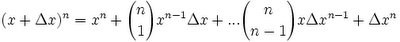(4) So a(x + Δx)n - axn, gives us: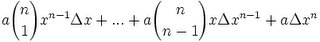(5) Then, dividing by Δx gives us: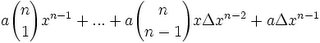(6) Finally, since Δx approaches 0, we can replace Δx with 0 to get: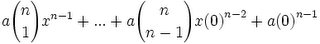(7) This then gives us: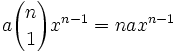QED

Example 1: (d/dx)(5x) = 5

f(x) = 5x

f'(x) = (d/dx)(5x) = d/dx(5x1) = (1)5x0 = 5.

Example 2: (d/dx)(1/x) = -1/(x2)

f(x) = 1/x

f'(x) = (d/dx)(1/x) = (d/dx)(x-1) = (-1)x-2 = -1/(x2)

Example 3: (d/dx)(4x2) = 8x

f(x) = 4x2

f'(x) = (d/dx)(4x2) = (4)(2)x1 = 8x

Lemma 3: Derivative of a Linear Combination

f(x) = a*g(x) + b*h(x) → f'(x) = a*g'(x) + b*h'(x)
where a,b are constants.

(1) f(x) = a*g(x) + b*h(x)

(2) f'(x) = f(x + Δx) - f(x) = a*g(x + Δx) + b*h(x + Δx) - [a*g(x) + b*h(x)] =

= a*[g(x+Δx) - g(x) ] + b*[h(x + Δx) - h(x)] = a*g'(x) + b*h'(x)

QED

Example 1: (d/dx)(4x + 5) = 4

f(x) = 4x + 5

f'(x) = (d/dx)(4x) + (d/dx)(5) = 4 + 0 = 4

Example 2: (d/dx)(2x2 + 3x) = 4x + 3

f(x) = 2x2 + 3x

f'(x) = (d/dx)(2x2) + (d/dx)(3x) = 4x + 3

Lemma 4: Product Rule: f(x) = g(x)h(x) → f'(x) = g(x)h'(x) + g'(x)h(x)
(1) Let f(x) = g(x)h(x)

(2) f'(x) = lim (Δx → 0) [ g(x+Δx)h(x + Δx) - g(x)h(x)]/Δx =

= lim(Δx → 0)[g(x + Δx)h(x+Δx)-g(x)h(x+Δx)+g(x)h(x+Δx)-g(x)h(x)]/Δx =

= lim(Δx→0)[g(x+Δx)h(x+Δx)-g(x)h(x+Δx)]/Δx +
lim(Δx→0)[g(x)h(x+Δx) - g(x)h(x)]/Δx =

= lim(Δx→0)[g(x+Δx)-g(x)]/Δx * lim(Δx→0)h(x+Δx) +
lim(Δx→0)g(x)*lim(Δx→0)[h(x+Δx)-h(x)]/Δx

(3) Now, we note that:
lim(Δx→ 0)g(x) = g(x)

lim(Δx→0)h(x+Δx) = h(x)

(4) We also note that:
lim(Δx→0)[g(x+Δx) - g(x)]/Δx = g'(x)

lim(Δx→0)[h(x+Δx)-h(x)]/Δx = h'(x)

(5) Combining #2, #3, and #4 gives us:
f'(x) = g'(x)h(x) + g(x)h'(x)

QED

Lemma 5: Reciprocal Rule

If f(x) is differentiable at x and f(x) ≠ 0 and u(x)=1/f(x)

Then:

u'(x) = -f'(x)/[f(x)]2

Proof:

(1) From the definition of the derivative and the definition of u(x), we have:

u'(x) = lim (Δx → 0) (1/Δx)[1/f(x+Δx) - 1/f(x)] =

= lim (Δx → 0)[f(x) - f(x + Δx)]/[Δx*f(x + Δx)*f(x)]

(3) Since:

[f(x) - f(x + Δx)]/[Δx*f(x + Δx)*f(x)] =(-1)*(1/[f(x+Δx)f(x)])*([f(x+Δx)-f(x)]/Δx),

we can use the Product Rule for Limits (see Lemma 2, here) to get:

lim (Δx → 0)[f(x) - f(x + Δx)]/[Δx*f(x + Δx)*f(x)] =

= lim (Δx → 0)(-1) * lim (Δx → 0)(1/[f(x+Δx)f(x)]) * lim (Δx → 0) ([f(x+Δx)-f(x)]/Δx)

(5) Since:

lim (Δx → 0)(-1) = -1.

lim (Δx → 0)(1/[f(x+Δx)f(x)]) = 1/[f(x)]2

lim (Δx → 0) ([f(x+Δx)-f(x)]/Δx) = f'(x)

We have:

u'(x) = (-1)*( 1/[f(x)]2)* f'(x) = -f'(x)/[f(x)]2

QED

Lemma 6: The Quotient Rule

If f(x) and g(x) are differentiable at x and g(x) ≠ 0, and u(x)=f(x)/g(x) with u(x) differentiable at x, then:

u'(x) = [f'(x)g(x) - f(x)g'(x)]/[g(x)]2

Proof:

(1) Using the definition for u(x), we have:

u(x) = f(x)/g(x) = f(x)*1/g(x)

(2) Using the Product Rule (see Lemma 4 above), we have:

u'(x) = f(x)*D[1/g(x)] + f'(x)*[1/g(x)]

(3) Using the Reciprocal Rule (see Lemma 5 above), we know that:

D[1/g(x)] = -g'(x)/[g(x)]2

(4) Applying step #3 to step #2 gives us:

u'(x) = f(x)*[-g'(x)/[g(x)]2] + f'(x)/g(x) = [-f(x)g'(x) + f'(x)g(x)]/[g(x)]2 = [f'(x)g(x) - f(x)g'(x)]/[g(x)]2

QEDAnonymous said...

Great blog. aptly named. Thank you very much. bookmarked:)

A small typo i wanted to point out, it should be Δx instead of x, i.e,
(Δy)/(Δx) where Δx is a value as small as possible. In mathematical terms, we are finding this ratio as Δx approaches a limit of 0.Anonymous said...

This is a Very good Blog! This is one of the most helpful articles that I have seen on the web and actually I think that this explains derivatives better than the Calculus book that I am using. Excellent job! Thanks!

Unknown said...